Latest Banking jobs   »   Quantitative Aptitude Quiz For IBPS PO...

# Quantitative Aptitude Quiz For IBPS PO Mains 2022-10th January

Q1. SBI bank has a free locker allowance for its customers but if any customer carries excess things it is charged at a constant rate per gm. The total charged paid by Veer & Mohit is Rs 110. If both Veer & mohit had carried twice the weight then, they actually did previously, then their charge would have been Rs 200 and Rs 100 respectively, Find charge of Veer?
(a) 100Rs
(b) 80Rs
(c) 160Rs
(d) 120Rs
(e) 180Rs

Directions (2-3): A and B invested Rs. (x – 1200) and Rs. (x + 800) in a partnership business. After one year A added Rs. 2000, while B withdraw Rs. 1600 and C joined them with Rs. (x + 2800). After two years the ratio of profit of A, B and C is 35 : 36 : 25.

Q2. Veer and Sameer invested Rs. (x + y) and Rs. (x + 2.5 y) in a business. If at the end of two years profit ratio of Veer and Sameer is 10 : 13. Then find value of 4.5y ?
(a) 8100 Rs.
(b) 8400 Rs.
(c) 7200 Rs.
(d) 9600 Rs.
(e) 10800 Rs.

Q3. P, Q and R invested in a business by making investment what A, B and C invested for second year respectively. P and Q invested for 8 months and 10 month respectively and profit share of P & Q &R is 32 : 32 : 25, then find for how many months R invested ?
(a) 3 months
(b) 1 months
(c) 11 months
(d) 5 months
(e) 8 months

Directions (4-5): Two vessels A & B contains (x + 24) l & (x + 60) l mixture respectively. Ratio of milk to water in vessel A is 7 : 5, while ratio of milk to orange juice in vessel B is 5 : 4. If 25% from vessel A & 50% from vessel B taken out so remaining milk in vessel A is 13 l more than that of remaining milk in vessel B.

Q4. If 50% from vessel A, 40% from vessel B taken out and mixed together, then find ratio of milk, water & orange in resulting mixture?
(a) 41 : 12 : 16
(b) 41 : 10 : 16
(c) 41 : 8 : 16
(d) 41 : 15 : 16
(e) 41 : 17 : 16

Q5. If vessel A & B poured together and X liter of water added in resulting mixture new ratio of milk, water and orange juice become 23 : 10 : 10. Find ‘X’?
(a) 15 l
(b) 12 l
(c) 10 l
(d) 20 l
(e) 8 l

Directions (6-7): A shopkeeper have two articles A & B. Marked price of article B is 20% more than marked price of article A, shopkeeper sold article A at 25% discount and article B at 20% discount, if he made 20% loss on article A and 6⅔% profit on article B and total loss of shopkeeper was Rs. 1785.

Q6. If shopkeeper want 25% profit on article A, then find at what price shopkeeper should sold the article?
(a) 16206.25 Rs.
(b) 16200. 25Rs.
(c) 16180.25 Rs.
(d) 16406.25 Rs.
(e) 16160.25 Rs.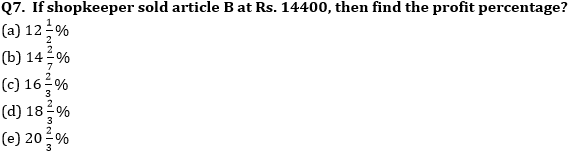Directions (8 -9): Shatish invested Rs. 1700 in a scheme ‘A’ for two years which offered S.I. annually at the rate of R% and gets total interest of Rs. 544. Another scheme B, which offered CI annually at the rate of (R – 6) %.

Q8. If a man invested Rs. (2000 + x) in scheme A for 2 years and Rs. (1600 + 3x) in scheme B for 2 years and interest got from scheme A is Rs180 more than interest got from scheme B, then find ‘x’?
(a) 800 Rs.
(b) 1000 Rs.
(c) 1600 Rs.
(d) 400 Rs.
(e) 2000 Rs.

Q9. A man invested an amount in the ratio of 3 : 2 at the rate of (R/2-0.5)% & (R – 8)% respectively. If bigger amount invested for two years and man got interest in the ratio of 15 : 16, then find smaller amount invested for how many years?
(a) 2 years
(b) 3 years
(c) 5 years
(d) 7 years
(e) 11 years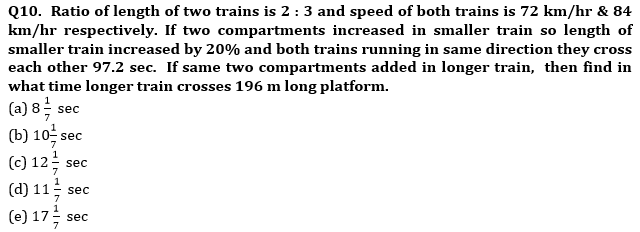Solutions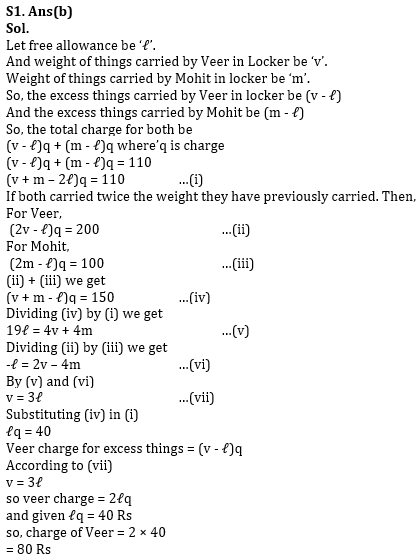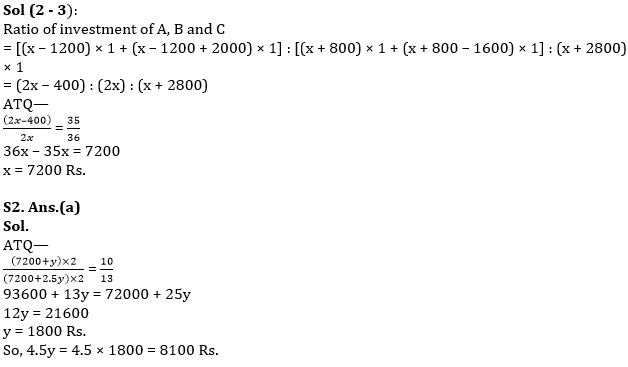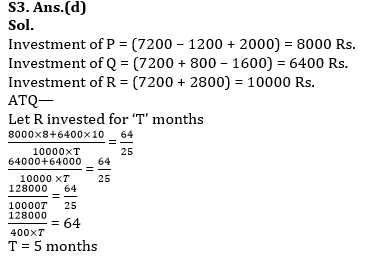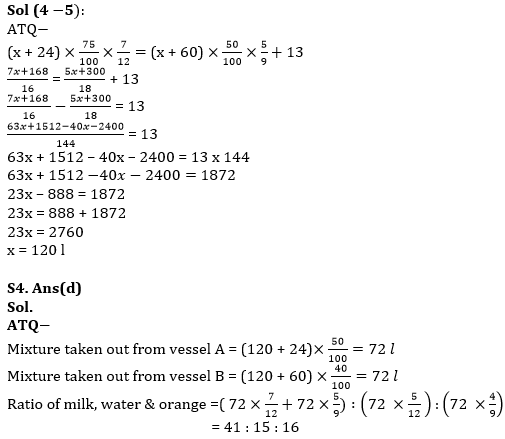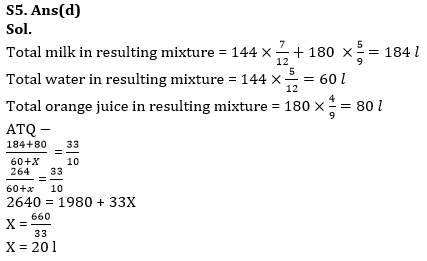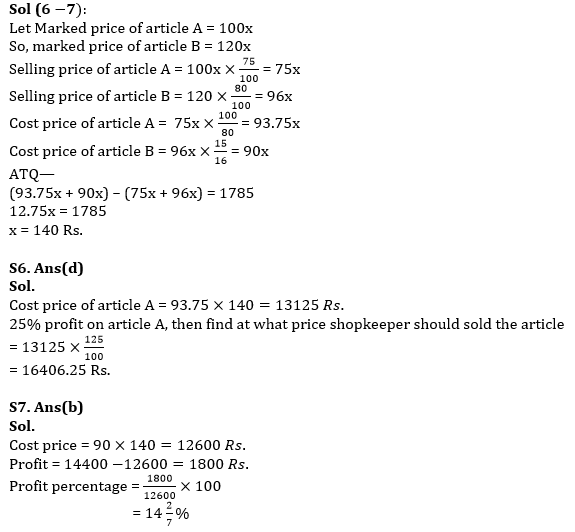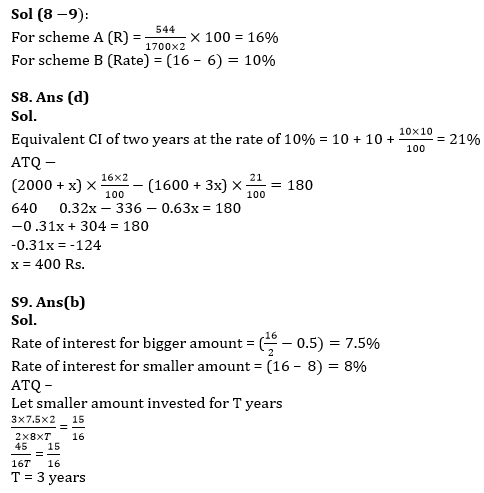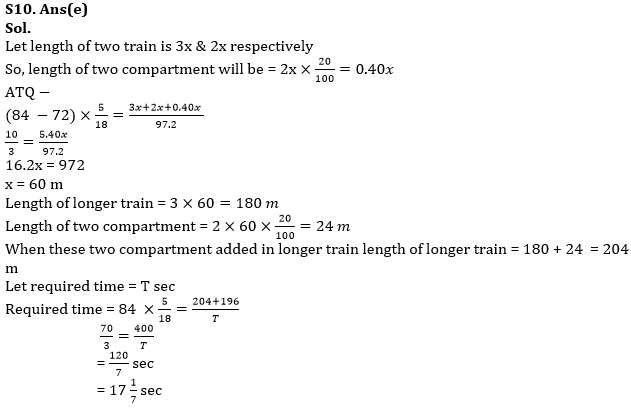#### Congratulations!Union Budget 2023-24: Free PDF